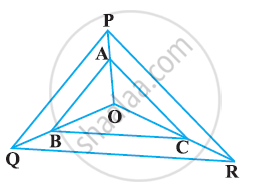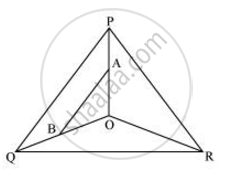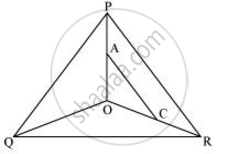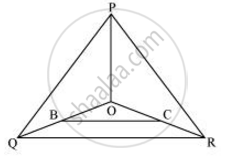# In the following figure, A, B and C are points on OP, OQ and OR respectively such that AB || PQ and AC || PR. Show that BC || QR. - Mathematics

In the following figure, A, B and C are points on OP, OQ and OR respectively such that AB || PQ and AC || PR. Show that BC || QR.#### SolutionIn Δ POQ, AB || PQ

:.(OA)/(AP) = (OB)/(BQ)   (basic proportionality theorem)  (i)In ΔPOR, AC||PR

:.(OA)/(AP) = (OC)/(CR)  (By basic proportionality theorem)  (ii)

From i and ii, we obtain

(OB)/(BQ) = (OC)/(CR)

:. BC || OQ (By Converse of basic proportionality theorem)Concept: Similarity of Triangles
Is there an error in this question or solution?

#### APPEARS IN

NCERT Class 10 Maths
Chapter 6 Triangles
Exercise 6.2 | Q 6 | Page 129